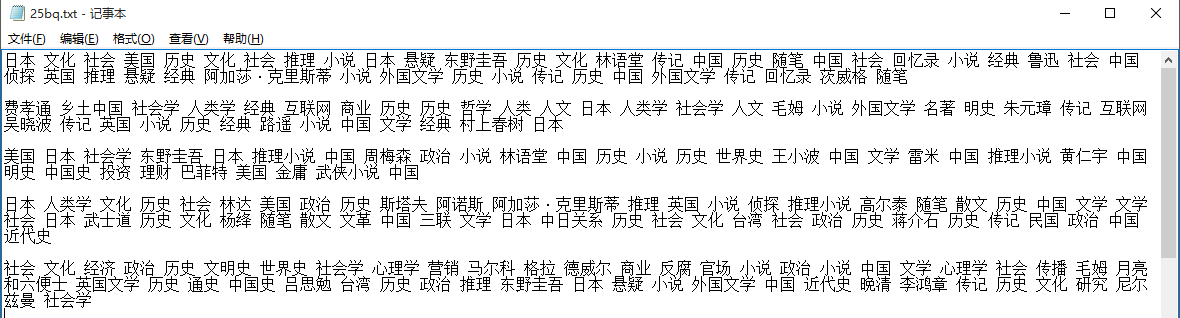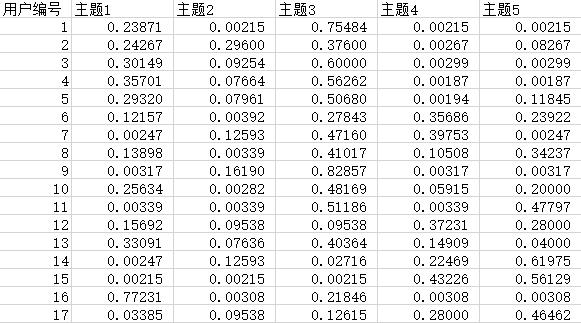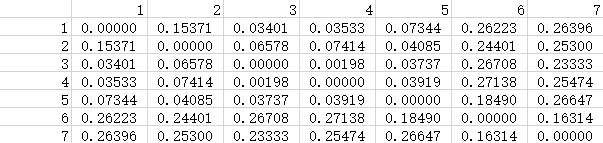# 利用python对中文文本数据进行LDA训练、计算概率距离

（代码来自于网络非原创，只是在此基础上修改,部分代码来自http://blog.csdn.net/eastmount/article/details/50891162

25名用户以及各自的标签全部保存在25bq.txt中1.读取需要进行训练的文本文件并进行LDA训练
（这里由于数据较少，所以设置主题k=5，迭代100次）

# -*- coding: utf-8 -*-
import numpy as np
import numpy
from sklearn.feature_extraction.text import CountVectorizer
import lda
import lda.datasets
if __name__ == "__main__":
#存储读取语料 一行预料为一个文档
corpus = []
for line in open('e:\\25bq.txt', 'r',encoding='utf-8').readlines():
#print line
corpus.append(line.strip())
#print corpus

#将文本中的词语转换为词频矩阵 矩阵元素a[i][j] 表示j词在i类文本下的词频
vectorizer = CountVectorizer()
x = vectorizer.fit_transform(corpus)
print(x)
analyze = vectorizer.build_analyzer()
weight = x.toarray()

model = lda.LDA(n_topics=5, n_iter=100,random_state=1)
model.fit(np.asarray(weight))     # model.fit_transform(X) is also available
topic_word = model.topic_word_    # model.components_ also works
#文档-主题（Document-Topic）分布
doc_topic = model.doc_topic_
a=doc_topic
numpy.savetxt('e:\\LDA\\100.csv', a, delimiter = ',') #将得到的文档-主题分布保存2.得到用户在其标签上的主题分布后，利用JS散度计算两两用户在主题概率间的距离

def KLD(P,Q):
return sum(p* log(p/q) for (p,q) in zip(P,Q))

def JSD_core(P,Q):
M = [0.5*(p+q) for (p,q) in zip(P,Q)]
return 0.5*KLD(P,M)+0.5*KLD(Q,M)

my_matrix = numpy.loadtxt(open("e:\\100.csv","rb"),delimiter=",",skiprows=0)
a=my_matrix

list1=[]
for i in range(0,135):
list2=[]
for j in range(0,135):
list2.append(JSD_core(a[i],a[j]))
list1.append(list2)
print(i)

f=open('e:\\100js.txt','w')
for i in list1:
k=' '.join([str(j) for j in i])
f.write(k+"\n")
f.close()